Real Numbers - Olympiad Level MCQ, Class 10 Mathematics

# Real Numbers - Olympiad Level MCQ, Class 10 Mathematics

Test Description

## 40 Questions MCQ Test | Real Numbers - Olympiad Level MCQ, Class 10 Mathematics

Real Numbers - Olympiad Level MCQ, Class 10 Mathematics for Class 10 2023 is part of Class 10 preparation. The Real Numbers - Olympiad Level MCQ, Class 10 Mathematics questions and answers have been prepared according to the Class 10 exam syllabus.The Real Numbers - Olympiad Level MCQ, Class 10 Mathematics MCQs are made for Class 10 2023 Exam. Find important definitions, questions, notes, meanings, examples, exercises, MCQs and online tests for Real Numbers - Olympiad Level MCQ, Class 10 Mathematics below.
Solutions of Real Numbers - Olympiad Level MCQ, Class 10 Mathematics questions in English are available as part of our course for Class 10 & Real Numbers - Olympiad Level MCQ, Class 10 Mathematics solutions in Hindi for Class 10 course. Download more important topics, notes, lectures and mock test series for Class 10 Exam by signing up for free. Attempt Real Numbers - Olympiad Level MCQ, Class 10 Mathematics | 40 questions in 40 minutes | Mock test for Class 10 preparation | Free important questions MCQ to study for Class 10 Exam | Download free PDF with solutions
 1 Crore+ students have signed up on EduRev. Have you?
Real Numbers - Olympiad Level MCQ, Class 10 Mathematics - Question 1

### Q. The greatest number which on dividing 1657 and 2037 leaves remainders 6 and 5 respectively, is:

Detailed Solution for Real Numbers - Olympiad Level MCQ, Class 10 Mathematics - Question 1

Required number = H.C.F. of (1657 - 6) and (2037 - 5)

= H.C.F. of 1651 and 2032 = 127.

Real Numbers - Olympiad Level MCQ, Class 10 Mathematics - Question 2

### The largest possible number with which when 60 and 98 are divided, leaves the remainder 3 in each case, is –

Real Numbers - Olympiad Level MCQ, Class 10 Mathematics - Question 3

### The largest possible number with which when 38, 66 and 80 are divided,leaves the remainder same is –

Detailed Solution for Real Numbers - Olympiad Level MCQ, Class 10 Mathematics - Question 3

Let the common remainder be  10

From the given numbers we now subtract the common remainder

i.e 38 − 10 = 28,66 −10 = 56, 80 −10 = 70

Now  we will find the HCF of 28, 56, 70

28 = 2 x 7 x 2

56 = 2 x 2 x 2 x 7

70 = 2 x 5 x 7

So HCF of 28, 56 and 70 = 2 x 7

So the required number is 14

Real Numbers - Olympiad Level MCQ, Class 10 Mathematics - Question 4

What is the least possible number which when divided by 24, 32 or 42 in each case it leaves the remainder 5?

Detailed Solution for Real Numbers - Olympiad Level MCQ, Class 10 Mathematics - Question 4

LCM of 24 , 32 & 42 = 672

The least possible number for your given case = 672 +5 = 677

Real Numbers - Olympiad Level MCQ, Class 10 Mathematics - Question 5

What is the least possible number which when divided by 24, 32 or 42 in each case leaves remainder 5. How many such numbers are possible between 666 and 8888?

Real Numbers - Olympiad Level MCQ, Class 10 Mathematics - Question 6

The least number which when divided by 5, 6 , 7 and 8 leaves a remainder 3, but when divided by 9 leaves no remainder, is:

Detailed Solution for Real Numbers - Olympiad Level MCQ, Class 10 Mathematics - Question 6

L.C.M. of 5, 6, 7, 8 = 840.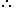Required number is of the form 840k + 3

Least value of k for which (840k + 3) is divisible by 9 is k = 2.Required number = (840 x 2 + 3) = 1683.

Real Numbers - Olympiad Level MCQ, Class 10 Mathematics - Question 7

What is the least possible number which when divided by 18, 35 or 42 leaves 2, 19, 26 as the remainders
respectively?

Detailed Solution for Real Numbers - Olympiad Level MCQ, Class 10 Mathematics - Question 7

N= 18a+2= 35b+19=42c+26

=> N+16= 18a=35b=42c

=> N+16 is divisible by LCM (18,35,42)

=> N+16 is divisible by 630 (=3*3*2*5*7)

=> N= 630k-16

=> Least possible number= 630*1-16= 614

Real Numbers - Olympiad Level MCQ, Class 10 Mathematics - Question 8

What is the least possible number which when divided by 2, 3, 4, 5, 6 leaves the remainders 1, 2, 3, 4, 5 respectively?

Detailed Solution for Real Numbers - Olympiad Level MCQ, Class 10 Mathematics - Question 8

Here,
2 - 1 = 1
3 - 2 = 1
4 - 3 = 1
5 - 4 = 1
6 - 5 = 1
That is, if we subtract 1 from the number divisible by 2, 3, 4, 5, 6, we get the required number.

The smallest number divisible by 2, 3, 4, 5, 6 will be the LCM of 2, 3, 4, 5, 6.

LCM of 2,3, 4, 5, 6 is 60
So, the required number which leaves a remainder of 1,2,3,4,5= L.C.M of (2,3,4,5,6)-1=59

Real Numbers - Olympiad Level MCQ, Class 10 Mathematics - Question 9

The least number which when divided by 2, 3, 4, 5 and 6 leaves the remainder 1 in each case. If the same number is divided by 7 it leaves no remainder. The number is

Detailed Solution for Real Numbers - Olympiad Level MCQ, Class 10 Mathematics - Question 9

The least common multiple of 2, 3, 4, 5, 6 is their LCM=60.
So, the answer is the least number such that it is a multiple of 7 and a multiple of 60 leaving remainder 1.

Real Numbers - Olympiad Level MCQ, Class 10 Mathematics - Question 10

How many numbers lie between 11 and 1111 which when divided by 9 leaves a remainder of 6 and when divided
by 21 leaves a remainder of 12?

Detailed Solution for Real Numbers - Olympiad Level MCQ, Class 10 Mathematics - Question 10

Number lies between 11 and 1111 When divide by 9 a remainder of 6 and when divided by 21 leave a remainder of 12 = 33, 96, 159 (all separated by 63)

a =33  d=63
1111=a+(n-1)63

1111=33+(n-1)63

1078=(n-1)63

1078/63=n-1

n=(1078+63)/63

n= 1141/63

n = 18.11

then total number=18

Real Numbers - Olympiad Level MCQ, Class 10 Mathematics - Question 11

The largest number which divides 70 and 125, leaving remainders 5 and 8 respectively, is

Detailed Solution for Real Numbers - Olympiad Level MCQ, Class 10 Mathematics - Question 11

Since, 5 and 8 are the remainders of 70 and 125, respectively. Thus, after subtracting these remainders from the numbers, we have the numbers 65 = (70-5),
117 = (125 – 8), which is divisible by the required number.
Now, required number = HCF of 65,117                 [for the largest number]
For this, 117 = 65 x 1 + 52 [∵ dividend = divisor x quotient + remainder]
⇒ 65 = 52 x 1 + 13
⇒ 52 = 13 x 4 + 0
∴ HCF = 13
Hence, 13 is the largest number which divides 70 and 125, leaving remainders 5 and 8.

Real Numbers - Olympiad Level MCQ, Class 10 Mathematics - Question 12

If x|y, where x > 0, y > 0 (x, y ε z) then –

Real Numbers - Olympiad Level MCQ, Class 10 Mathematics - Question 13

If a|b, then gcd of a and b is –

Detailed Solution for Real Numbers - Olympiad Level MCQ, Class 10 Mathematics - Question 13

Let a|b and the GCD(a,b) = m, then b=aq for some integer q and the GCD(a,b) can be expressed as a linear combination with some integer x and y,

ax + by = m .

Substituting in b we get,

ax + (aq)y = m

a(x + qy) = m

But x + qy = GCD(1,q) =1. Thus,

a(1) = m

Hence a = GCD(a,b), which is what we needed to show.

Real Numbers - Olympiad Level MCQ, Class 10 Mathematics - Question 14

A number 10x + y is multiplied by another number 10a + b and the result comes as 100p + 10q +r, where r = 2y, q = 2(x + y) and p = 2x; x, y < 5, q ≠ 0. The value of 10a + b may be:

Detailed Solution for Real Numbers - Olympiad Level MCQ, Class 10 Mathematics - Question 14

According to problem
(10x+y)(10a+b)=100p + 10q + r
By substituting the values
r=2y
q=(2x+y)
p=2x
(10x+y)(10a+b)=100*2x+10*2(x+y)+2y
(10x+y)(10a+b)=220x+22y
10a+b=22

Real Numbers - Olympiad Level MCQ, Class 10 Mathematics - Question 15

If x, y ε R and |x| + |y|= 0, then –

Real Numbers - Olympiad Level MCQ, Class 10 Mathematics - Question 16

If a, b, c ε R and a2 + b2 + c2 = ab + bc + ca, then –

Detailed Solution for Real Numbers - Olympiad Level MCQ, Class 10 Mathematics - Question 16

Consider,

a2 + b2 + c2 – ab – bc – ca = 0

Multiply both sides with 2, we get
2( a2 + b2 + c2 – ab – bc – ca) = 0
⇒ 2a+ 2b2 + 2c2 – 2ab – 2bc – 2ca = 0

⇒ a2 + a+ b2 + b+ c2 + c2– 2ab – 2bc – 2ca = 0
⇒ (a2 – 2ab + b2) + (b2 – 2bc + c2) + (c2 – 2ca + a2) = 0
⇒ (a – b)2 + (b – c)2 + (c – a)2 = 0
Since the sum of squares is zero, it means each term should be zero.
⇒ (a – b)2 = 0,  (b – c)2 = 0, (c – a)2 = 0
⇒ a – b = 0,  b – c = 0,  c – a = 0
⇒ a = b,  b = c, c = a
⇒ a = b = c

Real Numbers - Olympiad Level MCQ, Class 10 Mathematics - Question 17

If x, y ε R and x < y ⇒ x2 > y2 then –

Real Numbers - Olympiad Level MCQ, Class 10 Mathematics - Question 18

If x, y ε R and x > y ⇒ |x| > |y|, then–

Real Numbers - Olympiad Level MCQ, Class 10 Mathematics - Question 19

If x, y ε R and x > y ⇒ |x| < |y|, then–

Real Numbers - Olympiad Level MCQ, Class 10 Mathematics - Question 20

π and e are –

Detailed Solution for Real Numbers - Olympiad Level MCQ, Class 10 Mathematics - Question 20

π (Pi) and e are famous irrational numbers

π = 3.1415926535897932384626433832795... (and more)

e = 2.7182818284590452353602874713527 (and more ...)

We cannot write down a simple fraction that equals Pi.

The popular approximation of  22/7 = 3.1428571428571... is close but not accurate.

The first few terms of e add up to: 1 + 1 + 1/2 + 1/6 + 1/24 + 1/120 = 2.71666...

Real Numbers - Olympiad Level MCQ, Class 10 Mathematics - Question 21

If a, b ε R and a < b, then

Real Numbers - Olympiad Level MCQ, Class 10 Mathematics - Question 22

If x is a non-zero rational number and xy is irrational, then y must be –

Detailed Solution for Real Numbers - Olympiad Level MCQ, Class 10 Mathematics - Question 22

The product of a rational and irrational number is irrational.

2 × √5 = 2√5 is an irrational number.

Product of a rational and irrational number always irrational if rational number is not zero.

Real Numbers - Olympiad Level MCQ, Class 10 Mathematics - Question 23

The arithmetical fraction that exceeds it's square by the greatest quantity is –

Real Numbers - Olympiad Level MCQ, Class 10 Mathematics - Question 24

If x and y are rational numbers such that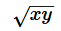is irrational, then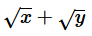is

Detailed Solution for Real Numbers - Olympiad Level MCQ, Class 10 Mathematics - Question 24

Take an example of 2 and 3.
When you multiply,
√2×√3=√6(Irrational).
√2+√3=Irrational.

Real Numbers - Olympiad Level MCQ, Class 10 Mathematics - Question 25

If x and y are positive real numbers, then –

Real Numbers - Olympiad Level MCQ, Class 10 Mathematics - Question 26

If two positive integers a and b are written as a = x3y2 and b = xy3; x, y are prime numbers, then HCF (a, b) is

Detailed Solution for Real Numbers - Olympiad Level MCQ, Class 10 Mathematics - Question 26

Given that, a = x3y2 = x x x x x x y x y
and b = xy3 = x x y x y x y
∴ HCF of a and b = HCF (x3y2, xy3) = x x y x y = xy2
[Since, HCF is the porduct of the smallest power of each common prime facter involved in the numbers]

Real Numbers - Olympiad Level MCQ, Class 10 Mathematics - Question 27

If x ε R, then |x|=

Real Numbers - Olympiad Level MCQ, Class 10 Mathematics - Question 28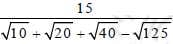is equal to –

Detailed Solution for Real Numbers - Olympiad Level MCQ, Class 10 Mathematics - Question 28

Explanation : 15/(10)1/2 + (20)1/2+ (40)1/2- (125)1/2

(3*5)/[(2)1/2(5)1/2 + 2(5)1/2 + 2(2)1/2(5)1/2 - 5(5)1/2]

= (3*5)/[3(2)1/2(5)1/2 - 3(5)1/2]

= (3*5)/3(5)1/2[(2)1/2 - 1]

= (5)1/2/[(2)1/2 - 1]

Rationalise it, we get

= (5)1/2/[(2)1/2 - 1] * [(2)1/2 + 1]/[(2)1/2 + 1]

= (5)1/2[(2)1/2 + 1]/(2 - 1)

= (5)1/2/[(2)1/2 + 1]

Real Numbers - Olympiad Level MCQ, Class 10 Mathematics - Question 29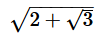+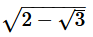is equal to –

Real Numbers - Olympiad Level MCQ, Class 10 Mathematics - Question 30

The expression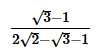is equal to –

Real Numbers - Olympiad Level MCQ, Class 10 Mathematics - Question 31

If x, y, z are real numbers such that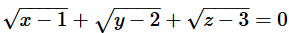then the values of x, y, z are respectively

Real Numbers - Olympiad Level MCQ, Class 10 Mathematics - Question 32

if a, b, c ε R and a > b ⇒ ac < bc, then –

Real Numbers - Olympiad Level MCQ, Class 10 Mathematics - Question 33

If a, b, c ε R and ac = bc ⇒ a = b, then –

Real Numbers - Olympiad Level MCQ, Class 10 Mathematics - Question 34

Between any two distinct rational numbers –

Detailed Solution for Real Numbers - Olympiad Level MCQ, Class 10 Mathematics - Question 34

Infinite number of rational numbers exist between any two distinct rational numbers. We know that a rational number is a number which can be written in the form of p/q​ where p and q are integers and q not equal to 0.

Real Numbers - Olympiad Level MCQ, Class 10 Mathematics - Question 35

The total number of divisors of 10500 except 1 and itself is –

Detailed Solution for Real Numbers - Olympiad Level MCQ, Class 10 Mathematics - Question 35

10,500 = 2^2 x 3^1 x 5^3 x 7^1

d(n) = (a + 1)(b + 1)(c + 1)(d + 1)

d(10500) = (2 + 1)(1 + 1)(3 + 1)(1 + 1)

d(10500) = (3)(2)(4)(2)

d(10500) = 48

Now 1 and 10500 should not be counted

so number of divisors = 48 - 2 = 46

Real Numbers - Olympiad Level MCQ, Class 10 Mathematics - Question 36

The sum of the factors of 19600 is –

Detailed Solution for Real Numbers - Olympiad Level MCQ, Class 10 Mathematics - Question 36

Properties of the number 19600

Factorization

-

2 * 2 * 2 * 2 * 5 * 5 * 7 * 7

Divisors- 1, 2, 4, 5, 7, 8, 10, 14, 16, 20, 25, 28, 35, 40, 49, 50, 56, 70, 80, 98, 100, 112, 140, 175, 196, 200, 245, 280, 350, 392, 400, 490, 560, 700, 784, 980, 1225, 1400, 1960, 2450, 2800, 3920, 4900, 9800, 19600

Count of divisors- 45

Sum of divisors - 54777

Real Numbers - Olympiad Level MCQ, Class 10 Mathematics - Question 37

The product of divisors of 7056 is –

Real Numbers - Olympiad Level MCQ, Class 10 Mathematics - Question 38

The number of odd factors (or divisors) of 24 is –

Detailed Solution for Real Numbers - Olympiad Level MCQ, Class 10 Mathematics - Question 38

24 can be divided by two odd numbers which are 1 and 3 only.

Real Numbers - Olympiad Level MCQ, Class 10 Mathematics - Question 39

The number of even factors (or divisors) of 24 is –

Detailed Solution for Real Numbers - Olympiad Level MCQ, Class 10 Mathematics - Question 39

Factors of 24 are 2, 3 ,4 6 8 12 and 24 itself
therefore , no of even factors are 6

Real Numbers - Olympiad Level MCQ, Class 10 Mathematics - Question 40

In how many ways can 576 be expressed as a product of two distinct factors?

Detailed Solution for Real Numbers - Olympiad Level MCQ, Class 10 Mathematics - Question 40

576 = 26 x 32
∴ Total number of factors = (6 + 1) (2 + 1) = 21
So the number of ways of expressing 576 as a product of two distinct factors =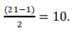Use Code STAYHOME200 and get INR 200 additional OFF Use Coupon Code
Information about Real Numbers - Olympiad Level MCQ, Class 10 Mathematics Page
In this test you can find the Exam questions for Real Numbers - Olympiad Level MCQ, Class 10 Mathematics solved & explained in the simplest way possible. Besides giving Questions and answers for Real Numbers - Olympiad Level MCQ, Class 10 Mathematics, EduRev gives you an ample number of Online tests for practice